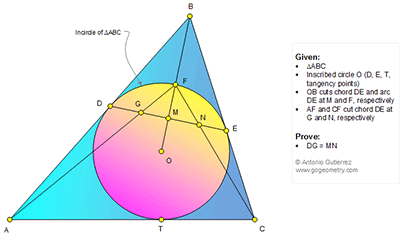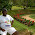Monday, February 20, 2017

Geometry Problem 1315: Triangle, Incircle, Tangent, Chord, Circle, Congruence

Geometry Problem. Post your solution in the comment box below.
Level: Mathematics Education, High School, Honors Geometry, College.

Details: Click on the figure below.1.Draw ⊥ to BM (extended) a) CC` meet BM at K b) AA` meet BM at L
Draw D`E` tg to O, // to DE, join D`to L (meet DE at G`), E` to K(meet DE atN`)
∆DD`G`~∆D`AL=> DG`/FM = AL/(FM+MK+KL) (1) ∆FGM~ ∆FAL=> GM/FM = AL/(FM+MK+KL)(2)
From (1) and (2) DG`=GM => DG`+GG`= GM +NN` => DG = MN

2.To C.t.e.o
https://goo.gl/photos/eH5HnBBBQcrkyQcEA
see link above for sketch per your solution. hope that it is correct
I agree with the first 3 lines of your solution.
In the last line, I am not sure how do you have DG'+GG'=GM+NN' . please explain
I note that given data " AC tangent to circle O " was never been used in your solution . please explain.

Peter Tran

3.To Peter
Your sketch is correct ( I can draw exactly in corel but I can`t post)
D`A=E`A` ∆BAA` isoceles, AL=LA` L midpoint, ∆D`DG`≡∆E`NE =>DG`=NE
∆D`KE` isoceles => GM=MN` from two conclusions we get GG`=NN` (DM=ME)
`` AC tg to O`` Important te go to the solution is to get similar triangles ,
isoceles triangles to get equal segments on DE, that is the key to go to the solution of P1317 I think every thing is clear now or you can ask again
because c.t.e.o. meaning is (C)ontributing (T)o (E)ach (O)ther

4.to c.t.e.o

I note that given data " AC tangent to circle O " was never been used in your solution .
Does it mean that the solution is correct for any position of A and C ? Please justify it
Peter Tran

5.To Peter
Pr need equal segment on the right and on the left of M, it need Three parallel lines and midpoints, which are D`E`, DE, AA`, and points M, K, L, so AA` need to be parallel to DE. So it is correct for every position of A and C ( see these conditions for P 1317).AC would be included just when ABC is isoceles or equilateral

1.https://goo.gl/photos/rjXc3exGocRKm9rQ7
correction due to typo in my previous comment.
For the purpose of discussion, let assume that point C move to point C1 ( see sketch).
AC1 will not tangent to circle O
K move to K1 , FC1 cut DE at N1.
All other points stay at the same locations.
DG will stay at the same length .
N will move to N1 closer to point E.
MN now become MN1 > MN.
Without going to detail analysis/proving ,by observation only we know for sure that position of N on DE is depend on position of C on BA’.
There is only one specific location of C on BA’ to make DG= MN.
I hope that Mr. Antonio Gutierrez or other geometry experts give us opinions about this subject.

Peter Tran

2.6.I think Peter's point is that AC can just as well cut the circle in 2 points rather than being tangent to it. Or it need not touch it at all.

Is the result still true then?

7.Yes it is true, but full conditions are DE, joining two tg points , and F (And P1317) midpoint of arc DE, but the way to achive result is what I was writing above ( my idea was to get same area => from same altitudes we get same base)
Prove : Draw three parallel lines, take some points on first line, take two points on third line (Or more but in equal distance). Join every point of first line with two points on third line => see segments on second line

8.If C goes to C1 then A goes to A1 and A1C1 tg to circle move: N on a new position and G on a new position but DG1=MN1 so AC must be tg to circle with two other conditions written above,( I was thinking for different kind of triangles ABC) but I can`t understand Incuding AC in solution

9.Could anyone please write a unified, coherent answer to this problem? Is has been riddled with typos, half explanations, corrections and so on. Please?
Jens Carstensen, Denmark

10.I think full explanation don`t help anybody as something left do .
I think first 3 lines are clear. Fill sketch of Peter: Extend CK to C` (C` on AB), extend AL to A` ( A` on BC). Join K to D` , join L to E`
From first 3 lines DG`= GM. At the same way on the right of M we get MN`= NE
From ∆CFC` isoceles => DG`=NE (1), from ∆AFA` isoceles => GM = MN`(2) from (1) and (2) and M midpoint add conclusions of first 3 lines we get GG`=NN`, DG`=MN`
or DG`+GG`=MN`+NN` => DG=MN
(There is something left, try)

11.https://goo.gl/photos/vvEvaHvhT5ebFUDy6
See below for the solution using geometry and trigonometry.
Let A, B, C are 3 angles of triangle ABC
Let R is the radius of incircle of triangle ABC
. Let u= ∠ (MFN) , v= ∠ (MFG), α= ∠ (MEF), x= ∠ (POQ)
Draw points K, L, P, Q , S and T per sketch
Note that F is the center of incircle of triangle DBE
O is the center of incircle of triangles ABC and TBS
So EF , OS and OC are angles bisectors of angles MEB , PSB and ACB respectively
So DF , OT and OA are angles bisectors of angles MDB , PTB and BAC respectively
We have EF// OS and α = ∠ (MEF)= ∠ (PSO)= 45- B/4
See below for detail calculation of OC , CK, OK, FK, CF, angle OCK , tan(u) , tan(v) in terms of R, α, x
OC= R/sin(α +x/2)
∠ (OCK)= α - x/2
CK= R.cos(α –x/2)/sin(α +x/2)
OK=R.sin(α -x/2)/sin(α +x/2)
FK= R.(sin(α +x/2)+sin(α -x/2))/sin(α +x/2)
CK=R.cos(α -x/2)/sin(α +x/2)
. tan(u)= CK/FK= cos(α-x/2)/(sin(α+x/2)+sin(α-x/2))
. tan(v)=AL/FL=cos(α+x/2)/( sin(α+x/2)+sin(α-x/2))
.tan(u)+tan(v)= 2.cos(α).cos(x/2)/(2.sin(α).cos(x/2))= 1/tan(α)…. (1)

But tan(u)= MN/MF
tan(v)= MG/MF
and 1/tan(α)= ME/MF
Replace these it in (1) we will have MN+MG= ME=MD
DG= DM-GM=ME-(GN-MN)
Replace ME=GN in above we have DG= MN

12.To Peter
Did you use given data AC tg to circle O, in your solution.
What do you think about 9 comment, (Prove)to be applied on this solution
My solution is not full, what is missing
What do you think if triangle would be isoceles, equilateral

13.To C.t.e.o

1. Yes , all given data of problem 1315 including " AC tangent to circle O" was used in my solution.
The solution indicate that tan(u)+ tan(v) is not depend on value of angle x or distance GN is independent to position of T on circle O.
2. Your solution/comments to this problem have many good points but it may not enough to get to the correct conclusion. Hope that you can provide more details in your solution including graphic so that others can understand it .I am sure that after reviewing , people can point out the missing points.

Peter Tran

14.I think Antonio must be having a pure geometry solution possibly using congruent triangles.

15.A conclusion from P1315, a key point to achive the solution
DG'=GM=MN'=NE (see triangles a)AGD and AFD' b) LMG' and LFD' linked to LM, LF)
I think there is nothing left

16.It took me a while but I finally found a way to prove it geometrically.
It has to do with tangential quadrilaterals, some of their properties are studied in previous problems.
K is the projection of C to line BM, and L is the projection of A to line BM.
Define HI parallel to DE, H in AB and I in AC.
J is the intersection of HC and AI.
Because of theorem of problem 153, J is in DE.
All the rest we need to do is to apply several triangle similarities with segments on parallel lines DE, CK and AL.
We have that:
DJ+JE=DE
2*AL*FM/FL + 2*CK*FM/FK=DE
GM+MN=DE/2=DG+GM
Then DG=MN

17.